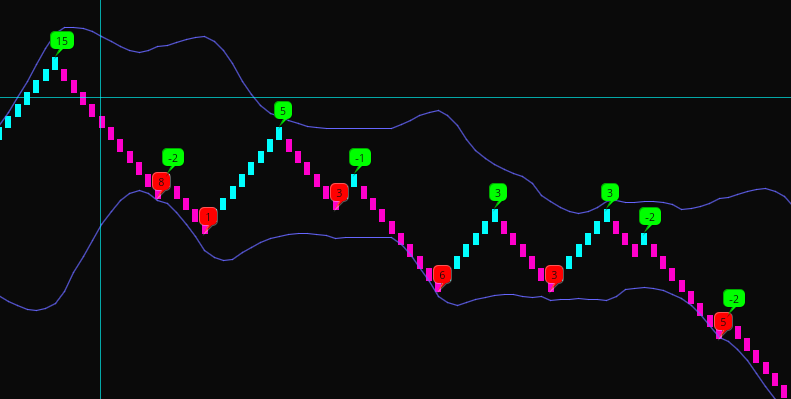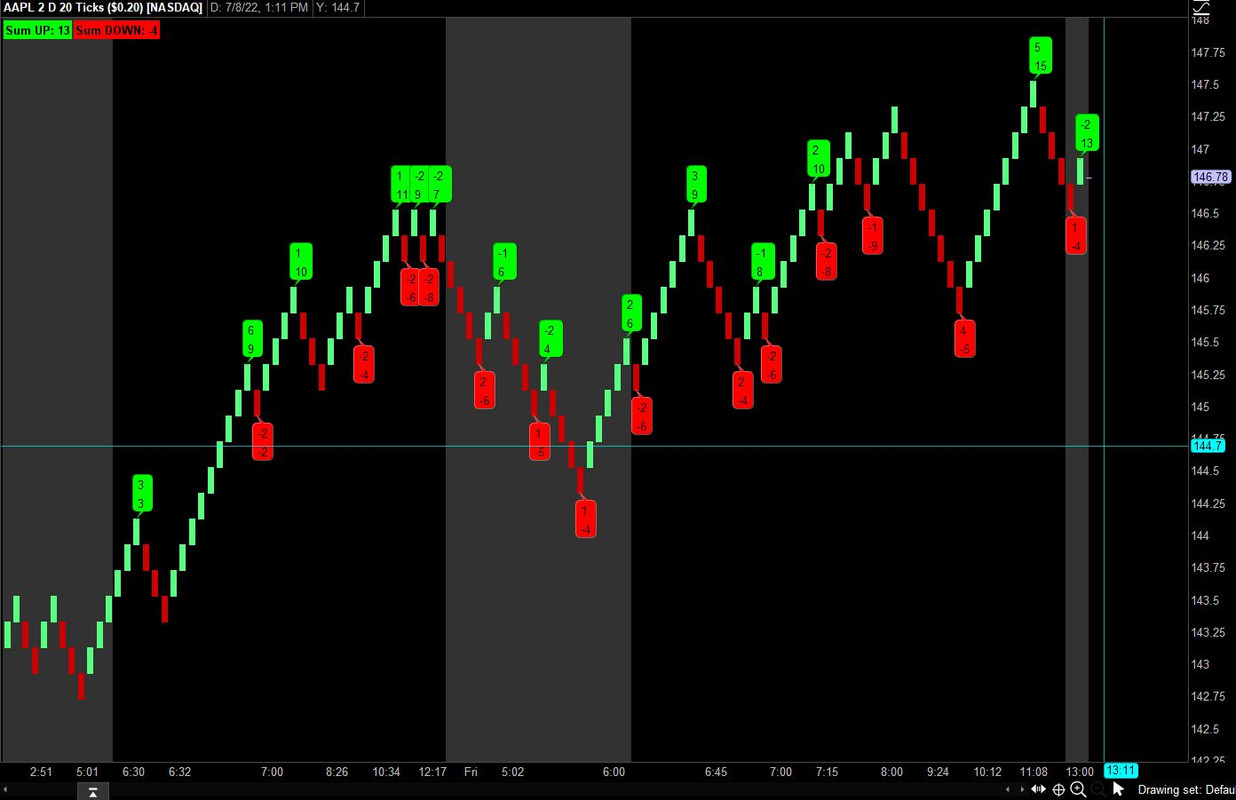# How do I add up all plotted values?

#### gomi111

##### New member
I am using Renko bars and made a bubble at the end of every run with a modified total count. How do I add up all the total values of all the bubbles? upFinal and downFinal separated if possible. Thanks

15090[/ATTACH]']Code:
``````#
#RENKO COUNT
#

def upBar = close > close;
def downBar = close < close;

def upStart = downBar and upBar;
def upCount = if upStart then 1 else if upBar then upCount + 1 else double.nan;
def upStop = if upBar and downBar then upCount else double.nan;
def upFinal = upStop - 3;

#def upSum = totalSum(upFinal);

def downStart = upBar and downBar;
def downCount = if downStart then 1 else if downBar then downCount + 1 else double.nan;
def downStop = if downBar and upBar then downCount else double.nan;
def downFinal = downStop - 3;

To simplify my question... How do add the previous value and current value of a variable?

#### Attachments

Last edited:
Solution
I am using Renko bars and made a bubble at the end of every run with a modified total count. How do I add up all the total values of all the bubbles? upFinal and downFinal separated if possible. Thanks

15094[/ATTACH]']Code:
``````#
#RENKO COUNT
#

def upBar = close > close;
def downBar = close < close;

def upStart = downBar and upBar;
def upCount = if upStart then 1 else if upBar then upCount + 1 else double.nan;
def upStop = if upBar and downBar then upCount else double.nan;
def upFinal = upStop - 3;

#def upSum = totalSum(upFinal);

def downStart = upBar and downBar;
def downCount = if downStart then 1...``````
I am using Renko bars and made a bubble at the end of every run with a modified total count. How do I add up all the total values of all the bubbles? upFinal and downFinal separated if possible. Thanks

15094[/ATTACH]']Code:
``````#
#RENKO COUNT
#

def upBar = close > close;
def downBar = close < close;

def upStart = downBar and upBar;
def upCount = if upStart then 1 else if upBar then upCount + 1 else double.nan;
def upStop = if upBar and downBar then upCount else double.nan;
def upFinal = upStop - 3;

#def upSum = totalSum(upFinal);

def downStart = upBar and downBar;
def downCount = if downStart then 1 else if downBar then downCount + 1 else double.nan;
def downStop = if downBar and upBar then downCount else double.nan;
def downFinal = downStop - 3;

To simplify my question... How do add the previous value and current value of a variable?

This displays the sum in bubbles and labelsRuby:
``````#
#RENKO COUNT
#

def upBar   = close > close;
def downBar = close < close;

def upStart = downBar and upBar;
def upCount = if upStart then 1 else if upBar then upCount + 1 else Double.NaN;
def upStop  = if upBar and downBar then upCount else Double.NaN;
def upFinal = upStop - 3;
def xup     = if !IsNaN(upFinal[-1]) then xup + upFinal[-1] else xup;
if xup== 0 then Double.NaN
else high,
upFinal[-1] + " \n" + xup, Color.GREEN);

AddLabel (yes, "Sum UP: " + xup, Color.green);

def downStart = upBar and downBar;
def downCount = if downStart then 1 else if downBar then downCount + 1 else Double.NaN;
def downStop  = if downBar and upBar then downCount else Double.NaN;
def downFinal = downStop - 3;
def xdn       = if !IsNaN(downFinal[-1]) then xdn + downFinal[-1] else xdn;
if xdn == 0 then Double.NaN
else low,
downFinal[-1] + "\n" + xdn, Color.RED, no);

addlabel(1, "Sum DOWN: " + xdn, color.red);``````

#### Attachments

•MerryDay

### Not the exact question you're looking for?

87k+ Posts
224 Online## The Market Trading Game Changer

Join 2,500+ subscribers inside the useThinkScript VIP Membership Club
• Exclusive indicators
• Proven strategies & setups
• Private Discord community
• Exclusive members-only content
• 1 full year of unlimited support

What is useThinkScript?

useThinkScript is the #1 community of stock market investors using indicators and other tools to power their trading strategies. Traders of all skill levels use our forums to learn about scripting and indicators, help each other, and discover new ways to gain an edge in the markets.

How do I get started?

We get it. Our forum can be intimidating, if not overwhelming. With thousands of topics, tens of thousands of posts, our community has created an incredibly deep knowledge base for stock traders. No one can ever exhaust every resource provided on our site.

If you are new, or just looking for guidance, here are some helpful links to get you started.

What are the benefits of VIP Membership?